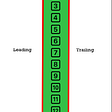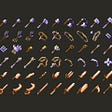# 1. Prepare the app

`func scene(_ scene: UIScene, willConnectTo session: UISceneSession, options connectionOptions: UIScene.ConnectionOptions) {        guard let winScene = (scene as? UIWindowScene) else { return }        window = UIWindow(windowScene: winScene)        window?.rootViewController = ViewController()        window?.makeKeyAndVisible()    }`

# 2. The UI elements

`private let taxCalculateContentView: UIView = {        let view = UIView()        view.backgroundColor = .blue        view.layer.cornerRadius = 20        view.clipsToBounds = true        view.translatesAutoresizingMaskIntoConstraints = false        return view    }()`
`private let titleLabel: UILabel = {        let label = UILabel()        label.text = "Value Added Tax"        label.textAlignment = .center        label.textColor = .systemBlue        label.font = UIFont.boldSystemFont(ofSize: 20)        label.translatesAutoresizingMaskIntoConstraints = false        return label    }()        private let amountTextField: UITextField = {        let textField = UITextField()        textField.backgroundColor = .white        textField.placeholder = "Amount / Net Price"        textField.borderStyle = .roundedRect        textField.keyboardType = .decimalPad        textField.textAlignment = .center        textField.translatesAutoresizingMaskIntoConstraints = false        return textField    }()        private let percentageTextField: UITextField = {        let textField = UITextField()        textField.backgroundColor = .white        textField.placeholder = "Tax in Percent"        textField.borderStyle = .roundedRect        textField.keyboardType = .numberPad        textField.textAlignment = .center        textField.translatesAutoresizingMaskIntoConstraints = false        return textField    }()        private let resultLabel: UILabel = {        let label = UILabel()        label.text = "Gross Price"        label.textAlignment = .center        label.textColor = .systemPink        label.font = UIFont.systemFont(ofSize: 20)        label.translatesAutoresizingMaskIntoConstraints = false        return label    }()`
`let calculateButton: UIButton = {        let button = UIButton(type: .system)        button.backgroundColor = .systemBlue        button.setTitle("Calculate", for: .normal)        button.tintColor = .white        button.layer.cornerRadius = 10        button.clipsToBounds = true        button.addTarget(self, action: #selector(calculateActionButton), for: .touchUpInside)        button.translatesAutoresizingMaskIntoConstraints = false        return button    }()`

# 3. SubViews and Auto Layout

`override func viewDidLoad() {        super.viewDidLoad()                taxCalculateContentView.addSubview(titleLabel)        taxCalculateContentView.addSubview(amountTextField)        taxCalculateContentView.addSubview(percentageTextField)        taxCalculateContentView.addSubview(resultLabel)        taxCalculateContentView.addSubview(calculateButton)                    view.backgroundColor = .systemBlue        view.addSubview(taxCalculateContentView)                setupAutoLayout()    }`
`func setupAutoLayout() {        taxCalculateContentView.leftAnchor.constraint(equalTo: view.leftAnchor, constant: 20).isActive = true        taxCalculateContentView.rightAnchor.constraint(equalTo: view.rightAnchor, constant: -20).isActive = true        taxCalculateContentView.heightAnchor.constraint(equalToConstant: view.frame.height / 3).isActive = true        taxCalculateContentView.centerYAnchor.constraint(equalTo: view.centerYAnchor).isActive = true                titleLabel.topAnchor.constraint(equalTo: taxCalculateContentView.topAnchor, constant: 10).isActive = true        titleLabel.leftAnchor.constraint(equalTo: taxCalculateContentView.leftAnchor, constant: 40).isActive = true        titleLabel.rightAnchor.constraint(equalTo: taxCalculateContentView.rightAnchor, constant: -40).isActive = true        titleLabel.heightAnchor.constraint(equalToConstant: 40).isActive = true                amountTextField.topAnchor.constraint(equalTo: titleLabel.bottomAnchor, constant: 10).isActive = true        amountTextField.leftAnchor.constraint(equalTo: taxCalculateContentView.leftAnchor, constant: 40).isActive = true        amountTextField.rightAnchor.constraint(equalTo: taxCalculateContentView.rightAnchor, constant: -40).isActive = true        amountTextField.heightAnchor.constraint(equalToConstant: 40).isActive = true        percentageTextField.topAnchor.constraint(equalTo: amountTextField.bottomAnchor, constant: 20).isActive = true        percentageTextField.leftAnchor.constraint(equalTo: taxCalculateContentView.leftAnchor, constant: 60).isActive = true        percentageTextField.rightAnchor.constraint(equalTo: taxCalculateContentView.rightAnchor, constant: -60).isActive = true        percentageTextField.heightAnchor.constraint(equalToConstant: 40).isActive = true        resultLabel.topAnchor.constraint(equalTo: percentageTextField.bottomAnchor, constant: 20).isActive = true        resultLabel.leftAnchor.constraint(equalTo: taxCalculateContentView.leftAnchor, constant: 60).isActive = true        resultLabel.rightAnchor.constraint(equalTo: taxCalculateContentView.rightAnchor, constant: -60).isActive = true        resultLabel.heightAnchor.constraint(equalToConstant: 40).isActive = true        calculateButton.topAnchor.constraint(equalTo: resultLabel.bottomAnchor, constant: 20).isActive = true        calculateButton.leftAnchor.constraint(equalTo: taxCalculateContentView.leftAnchor, constant: 40).isActive = true        calculateButton.rightAnchor.constraint(equalTo: taxCalculateContentView.rightAnchor, constant: -40).isActive = true        calculateButton.heightAnchor.constraint(equalToConstant: 40).isActive = true    }`

# 4. UIButton Action and Summary

`@objc func calculateActionButton(sender: UIButton!) {        let amount = amountTextField.text?.replacingOccurrences(of: ",", with: ".")        let percentage = percentageTextField.text                if !amount!.isEmpty && !percentage!.isEmpty {            let result = Double(amount!)! * (1 + (Double(percentage!)! / 100))            resultLabel.text = "Gross Price: \(String(format: "%.2f", result))"        }    }`

--

--

--

## More from David Koenig

Swift (iOS, iPadOS & macOS) Developer

Love podcasts or audiobooks? Learn on the go with our new app.

## Scroll to Different Positions in a ScrollView With ScrollViewReader in SwiftUI 2## Tic Toc: What Timer Is It?## Data Structures and Algorithms in Swift: Arrays## Update on #Hyprr with an overview of a new Video Preview feature and the updated AI.## Inheriting existing Class type to support ObservableObject## David Koenig

Swift (iOS, iPadOS & macOS) Developer

## Get your weapon of choice with Swift## Quickstart guide for Native Mobile App Automation On Mac M1/M1Pro Using Appium## Supporting Accessibility in your iOS app## How to build iOS Authentication with Firebase Part II Sign Up User 2022